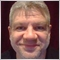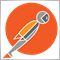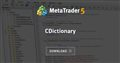# OOP issue : idea to break a vicious circle ? - page 242646

lippmaje:

How about processing the generic value with a function array.

And yes, you could build a 'custom' union to hold more complex types.

Yes I thought about the function pointer usage but didn't explore it yet.

I will keep you posted later, thanks for all.2692

https://www.mql5.com/en/code/19314CDictionary
• www.mql5.com
An implementation of the dictionary (associative array) data structure in MQL5, based on CArrayObj and CList. Hash Function Usage It can also store pointers to objects (but not objects or structures): Collision Handling Each entry would have its own hash. If two or more entries share the same hash, entries are compared by key and typename. This...42646

nicholi shen:
https://www.mql5.com/en/code/19314
Using an index or a key is not the problem. This solve nothing related to this topic.1385

Not perfect but maybe this can help.
I updated because the template is available in struct, as opposed to what I've said earlier.

```struct MyMqlParam : public MqlParam
{
public:
ulong             ulong_value;
template<typename T>
bool              Set(T value);
};
//+------------------------------------------------------------------+
//|                                                                  |
//+------------------------------------------------------------------+
template<typename T>
bool MyMqlParam::Set(T value)
{
if(typename(value)=="string")
{
this.type=TYPE_STRING;
this.string_value=(string)value;
}
else if(typename(value)=="int")
{
this.type=TYPE_INT;
this.integer_value=(long)value;
}
else if(typename(value)=="double")
{
this.type=TYPE_DOUBLE;
this.double_value=(double)value;
}
else if(typename(value)=="bool")
{
this.type=TYPE_BOOL;
this.integer_value=(int)value;
}
else if(typename(value)=="datetime")
{
this.type=TYPE_DATETIME;
this.integer_value=(datetime)value;
}
else if(typename(value)=="color")
{
this.type=TYPE_COLOR;
this.integer_value=(color)value;
}
else if(typename(value)=="long")
{
this.type=TYPE_LONG;
this.integer_value=(long)value;
}
else if(typename(value)=="ulong")
{
this.type=TYPE_ULONG;
this.ulong_value=(ulong)value;
}
else if(typename(value)=="uint")
{
this.type=TYPE_UINT;
this.integer_value=(uint)value;
}
else
{
this.type=TYPE_INT;
this.integer_value=(int)value;
}
return true;
}
//+------------------------------------------------------------------+
//|                                                                  |
//+------------------------------------------------------------------+
void OnStart()
{
MyMqlParam arr;
MyMqlParam p;
for(int i=0;i<ArraySize(arr);i++)
{
p.type=(ENUM_DATATYPE)MathMod(MathRand(),14);
switch(p.type)
{
case TYPE_BOOL:
p.Set<bool>(i);
break;
case TYPE_CHAR:
p.Set<char>(i);
break;
case TYPE_UCHAR:
p.Set<uchar>(i);
break;
case TYPE_SHORT:
p.Set<short>(i);
break;
case TYPE_USHORT:
p.Set<ushort>(i);
break;
case TYPE_COLOR:
p.Set<color>(i);
break;
case TYPE_INT:
p.Set<int>(i);
break;
case TYPE_UINT:
p.Set<uint>(i);
break;
case TYPE_DATETIME:
p.Set<datetime>(iTime(NULL,PERIOD_CURRENT,i));
break;
case TYPE_LONG:
p.Set<long>(i);
break;
case TYPE_ULONG:
p.Set<ulong>(i);
break;
case TYPE_FLOAT:
p.Set<float>(i);
break;
case TYPE_DOUBLE:
p.Set<double>(i);
break;
case TYPE_STRING:
p.Set<string>(i);
break;
}
arr[i]=p;
}

for(int i=0;i<ArraySize(arr);i++)
{
p=arr[i];
switch(p.type)
{
case TYPE_BOOL:
Print("val at ",i," is of ",EnumToString(p.type)," = ",(bool)p.integer_value);
break;
case TYPE_CHAR:
Print("val at ",i," is of ",EnumToString(p.type)," = ",(char)p.integer_value);
break;
case TYPE_UCHAR:
Print("val at ",i," is of ",EnumToString(p.type)," = ",(uchar)p.integer_value);
break;
case TYPE_SHORT:
Print("val at ",i," is of ",EnumToString(p.type)," = ",(short)p.integer_value);
break;
case TYPE_USHORT:
Print("val at ",i," is of ",EnumToString(p.type)," = ",(ushort)p.integer_value);
break;
case TYPE_COLOR:
Print("val at ",i," is of ",EnumToString(p.type)," = ",(color)p.integer_value);
break;
case TYPE_INT:
Print("val at ",i," is of ",EnumToString(p.type)," = ",(int)p.integer_value);
break;
case TYPE_UINT:
Print("val at ",i," is of ",EnumToString(p.type)," = ",(uint)p.integer_value);
break;
case TYPE_DATETIME:
Print("val at ",i," is of ",EnumToString(p.type)," = ",(datetime)p.integer_value);
break;
case TYPE_LONG:
Print("val at ",i," is of ",EnumToString(p.type)," = ",(long)p.integer_value);
break;
case TYPE_ULONG:
Print("val at ",i," is of ",EnumToString(p.type)," = ",(ulong)p.integer_value);
break;
case TYPE_FLOAT:
Print("val at ",i," is of ",EnumToString(p.type)," = ",(float)p.double_value);
break;
case TYPE_DOUBLE:
Print("val at ",i," is of ",EnumToString(p.type)," = ",(double)p.double_value);
break;
case TYPE_STRING:
Print("val at ",i," is of ",EnumToString(p.type)," = ",(string)p.string_value);
break;
}
}
}```42646

Amir Yacoby:

Not perfect but maybe this can help.
There is no template for structures - It compiles but not run. So, I don't use the Set method, just left it there in case you might be interested to wrap it in a class.

Thanks for your answer. Of course enumerating each type would work, but it's what I want to avoid. You can imagine my real code than to just get or print the value.42646

nicholi shen:

From original post :

So first question : is there a better solution than a factory method and an ENUM ?

1. The data comes from a text file, they are string, it's a requirement. So you can't do that :

```   dict.Set(KEY, 1.23);
dict.Set(KEY, "Hello");
dict.Set(KEY, 40);```

Example, imagine I have to call a functionA with an int parameter, or a functionB with a double parameter.

I will need to declare a variable for any type, then call the "dynamic cast" function, trying one by one :

2. Your code does the same as the code I posted, the details are just hidden in a dictionary. Only difference, when you need to use the values you are using a "typename", which is just similar to "switch" or "if". You still need to enumerate all types, which is what I am trying to avoid.

3. @lippmaje understood well the problem, so I guess what I want is correctly articulated. Feel free to ask question about what is not clear for you.

4. Please stop your sarcasms and your provocative attitude, if you can't, do me a favor : ignore me.3064

Did you try the CArrayObj way ? It is super generic, super dynamic and super convenient.
You can make collections of collections.
Possibilities in term of data organization are so numberous that it might help in your case.
https://www.mql5.com/en/docs/standardlibrary/datastructures/carrayobj197

Alain Verleyen:

Hello,

So, if I understand it correctly you have the file with entries like "INT:1234" and "DOUBLE:3.15" and so on and you do want to create a vector with them - i.e. an array compromised of various types. Then, you are saying you do want to know what type sits in each vector position so yo9u can use it in a e.g. proper function. So, some remarks from me:

a) Why creating a vector in the first place and not create instead all the wimple arrays you want ? Maybe this is "circle" you are referring to, maybe you do not want that compound vector

b) OK, let 's say you do need that compound vector (which by the way will be slower than simple arrays), but next you have to know the type on each position ; first of all, I am not sure MQL has that knowledge either; MetaQuotes could very well only store the necessary information concerning the size on each entry on the vector - and that only to use it for IO operations on that vector anyway; so it is maybe up to us to keep a record of what is going on in the vector. So I would propose to create a simple array along with the vector and each time you create an entry in the vector create a corresponding entry in the array which would tell the kind of type that exists in the vector - normally an array with an enum type - for visibility

greets42646

Demos Stogios:

Hello,

So, if I understand it correctly you have the file with entries like "INT:1234" and "DOUBLE:3.15" and so on and you do want to create a vector with them - i.e. an array compromised of various types. Then, you are saying you do want to know what type sits in each vector position so yo9u can use it in a e.g. proper function. So, some remarks from me:

a) Why creating a vector in the first place and not create instead all the wimple arrays you want ? Maybe this is "circle" you are referring to, maybe you do not want that compound vector

b) OK, let 's say you do need that compound vector (which by the way will be slower than simple arrays), but next you have to know the type on each position ; first of all, I am not sure MQL has that knowledge either; MetaQuotes could very well only store the necessary information concerning the size on each entry on the vector - and that only to use it for IO operations on that vector anyway; so it is maybe up to us to keep a record of what is going on in the vector. So I would propose to create a simple array along with the vector and each time you create an entry in the vector create a corresponding entry in the array which would tell the kind of type that exists in the vector - normally an array with an enum type - for visibility

greets

The circle I am referring to is the following, if you want to create generic code (in Stepanov sense), you use templates. Then if you want to create dynamic and flexible code (so a code where the minimum "stuff" are hard coded and fixed at compile time, but rather at run time), you will use polymorphism. When you try to combine that you have "problems", templates are static and limit the "polymorphism" of your code.

I could use typed arrays but that's at the opposite of my purpose, and is just moving the problem somewhere else. I talked about vectors, someone proposed dictionary, you arrays, it's all fine.

The problem with your proposal to use an array beside the vector is an other way similar to what I use, you will still have the problem to match your ENUM and mql types. So enumerate and repeat code. Probably what I want to achieve is not doable in mql and I will have to make some compromises.